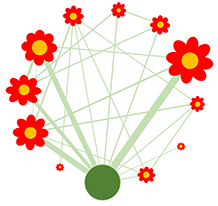We will use different data to illustrate Missing Completely At Random (MCAR), Missing At Random (MAR), and Missing Not At Random. First, we will present the three missingness situations, and afterwards, we show some tools to analyse the missingness patterns. There will be some redundancy of the plots used, but so the reader can chose the preferred tool.

Here you see a list of the packages we use for these analyses (click on the code button if you don’t see the black boxes with the code).

This page is still work in progress, this is the version from 13-01-2022. If you want to report an error, you can do this here Click to send an e-mail to Roger Hilfiker.

``````knitr::opts_chunk\$set(echo = TRUE)
r <- getOption("repos")
r["CRAN"] <- "https://stat.ethz.ch/CRAN/"
options(repos = r)
list.of.packages <- c("bookdown","rmarkdown" ,"knitr","rio", "psych","janitor",
"tidyverse","jtools","summarytools", "qgraph",  "gtsummary" , "viridis", "wesanderson", "missMethods", "ggpubr", "ggrepel", "naniar", "finalfit", "missMethods", "rpart", "rpart.plot")
new.packages <- list.of.packages[!(list.of.packages %in% installed.packages()[,"Package"])]
if(length(new.packages)) install.packages(new.packages)

library(summarytools)
library(psych)
library(janitor)
library(sjlabelled)
library(tidyverse)
library(gtsummary)
library(viridis)
library(wesanderson)
library(missMethods)
library(ggpubr)
library(ggrepel)
library(naniar)
library(finalfit)
library(rpart)
library(rpart.plot)
library(mice)
library(knitr)``````

# 1 Missing Completely at Random (MCAR)

We use some data from a published study and then we delete randomly some data.
This downloaded dataset has no missing values. So we will present the analysis without the missing values. Then, we will delete some values: We can simulate Missing Completely at Random values with the package missMethods, see here for a tutorial:.

Load the data directly from the web See the article here:

``````df1<-rio::import("https://doi.org/10.1371/journal.pone.0262238.s008", format="xlsx")
df1<-df1 %>%
rename(WalkingDistance_m_6min=Distance30) %>%
select(Number, ActiveSmoking, Age, Sex, COPDduration,FEV1, WalkingDistance_m_6min )``````

### 1.0.1 Dataset without missing values

Below you see a summary of the data, here still without missing data:

``````options(width = 300)
summary(df1)``````
``````##      Number      ActiveSmoking        Age             Sex        COPDduration        FEV1       WalkingDistance_m_6min
##  Min.   : 1.00   Min.   :  0.0   Min.   :53.00   Min.   :1.00   Min.   : 1.00   Min.   :0.440   Min.   :214.7
##  1st Qu.:14.25   1st Qu.:  0.0   1st Qu.:64.00   1st Qu.:1.00   1st Qu.: 7.25   1st Qu.:1.015   1st Qu.:304.8
##  Median :27.50   Median :  0.0   Median :68.50   Median :1.00   Median :16.00   Median :1.540   Median :371.4
##  Mean   :28.10   Mean   :160.1   Mean   :69.08   Mean   :1.08   Mean   :25.40   Mean   :1.496   Mean   :359.8
##  3rd Qu.:41.75   3rd Qu.:  1.0   3rd Qu.:76.00   3rd Qu.:1.00   3rd Qu.:44.25   3rd Qu.:1.805   3rd Qu.:408.0
##  Max.   :56.00   Max.   :999.0   Max.   :80.00   Max.   :2.00   Max.   :72.00   Max.   :2.530   Max.   :555.3``````

### 1.0.2 Scatterplot FEV1 - Six Minute Walking Distance (no missing data)

We plot the association between FEV1 and six minute walking distance:

``````correlation_Sex1<-cor(df1[df1\$Sex==1,6:7])
correlation_Sex2<-cor(df1[df1\$Sex==2,6:7])

ggplot(data=df1, aes(FEV1, WalkingDistance_m_6min, group=factor(Sex), colour=factor(Sex)))+
geom_point()+
``````